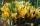Fill letters instead of digits so the indicated sum (equal letters represent equal digits). What number is hidden under the letter J?

A
A H
A H O
A H O J
--------------------------
4 3 2 1

Result

J =  1

Solution:Leave us a comment of this math problem and its solution (i.e. if it is still somewhat unclear...):Be the first to comment!To solve this verbal math problem are needed these knowledge from mathematics:

Do you have a linear equation or system of equations and looking for its solution? Or do you have quadratic equation?

Next similar math problems:

1. Roman numerals 2+Add up the number writtens in Roman numerals. Write the results as a roman numbers.
2. SummandOne of the summands is 145. The second is 10 more. Determine the sum of the summands.
3. CrocusesThe garden grow daffodils, crocuses and roses. 1400 daffodils, crocuses is 462 more and roses is 156 more than crocuses. How many are all the flowers in the garden?
4. Number unknownAdela thought the two-digit number, she added it to its ten times and got 407. What number does she think?
5. Number with onesThe first digit of the number is 1, if we move this digit to the end we get a 3 times higher number, which is the number?
6. Unknown number 11That number increased by three equals three times itself?
7. Last pageTwo consecutive sheets dropped out of the book. The sum of the numbers on the sides of the dropped sheets is 154. What is the number of the last page of the dropped sheets?
8. I thinkI think a number. When I multiply it by five, and after that I subtract 477, I get the same number as if I multiplied it twice. What number do I think?
9. InfirmaryTwo thirds of children from the infirmary went on a trip seventh went to bathe and 40 children remained in the gym. How many children were treated in the infirmary?
10. Dropped sheetsThree consecutive sheets dropped from the book. The sum of the numbers on the pages of the dropped sheets is 273. What number has the last page of the dropped sheets?
11. Simple equation 8Solve the following equation: 36=-(1+7x)-6(-7-x)
12. Find xSolve: if 2(x-1)=14, then x= (solve an equation with one unknown)
13. PopsiclesFrancis went to buy ice lollies. If he buy 8 popsicles he missed 4 USD. When he buy 7 popsicles, got back 1 USD. How many USD was a popsicle?
14. Simple equationSolve the following simple equation: 2. (4x + 3) = 2-5. (1-x)
15. CagesHonza had three cages (black, silver, gold) and three animals (guinea pig, rat and puppy). There was one animal in each cage. The golden cage stood to the left of the black cage. The silver cage stood on the right of the guinea pig cage. The rat was in the
16. If-then equationIf 5x - 17 = -x + 7, then x =
17. Negative in equation2x + 3 + 7x = – 24, what is the value of x?